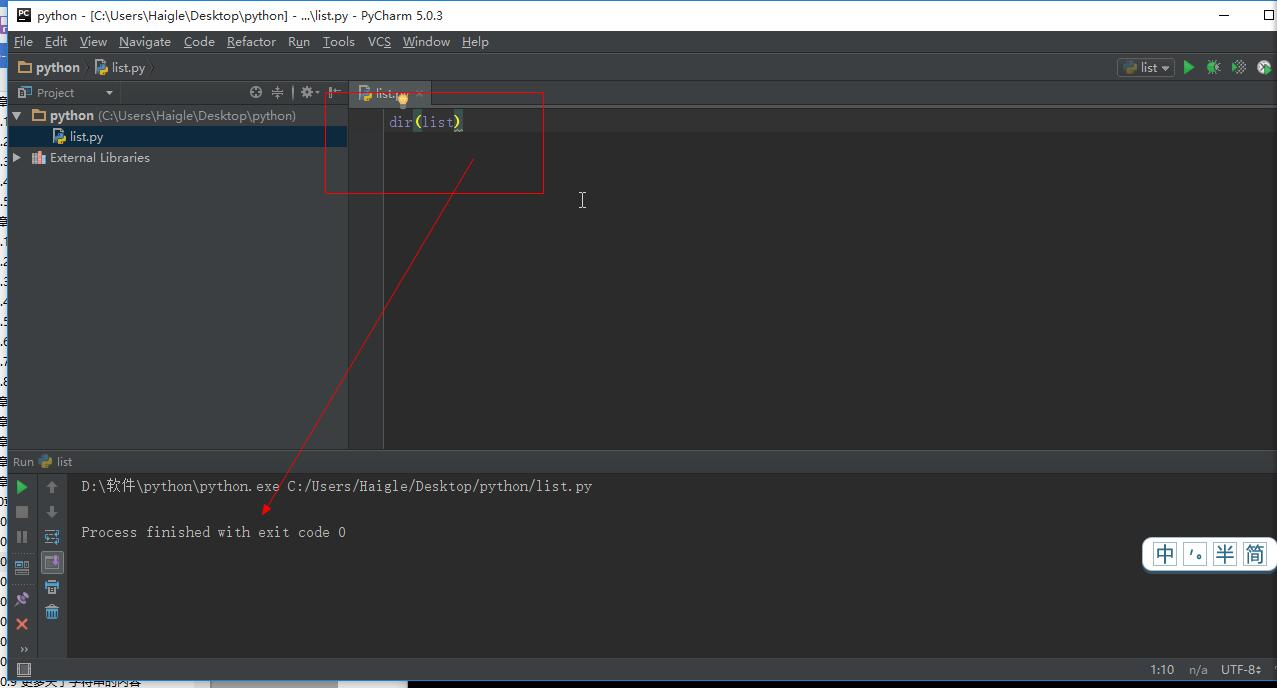1个回答Python+OpenCV计算机视觉

Python+OpenCV计算机视觉

#文件random_walk.py from random import choice class Randomwalk(): def _ _init_ _ (self, num_points=500): """初始化随机漫步的属性""" self.num_points = num_points # 所有随机漫步都始于（0,0） self.x_values =  self.y_values =  def fill_walk(self): """计算随机漫步所包含的所有点” """ # 不断漫步， 直到列表达到指定的长度 while len(self.x_values) < self.num_points: # 决定前进方向以及沿着个方向前进的距离 x_direction = choice([1, -1]) x_distance = choice([0, 1, 2, 3, 4]) x_step = x_direction * x_distance y_direction = choice([1, -1]) y_distance = choice([1, 2, 3, 4]) y_step = y_direction * y_distance # 拒绝原地踏步 if x_step == 0 and y_step == 0: continue # 计算下一个点的x值和y值 next_x = self.x_values[-1] + x_step next_y = self.y_values[-1] + y_step self.x_values.append(next_x) self.y_values.append(next_y) #文件rw_visual.py import matplotlib.pyplot as plt from random_walk import Randomwalk rw = Randomwalk() rw.fill_walk() plt.scatter(rw.x_values, rw.y_values, s=15) plt.show() 在idle里面能出结果，在pycharm中没报错，但没结果

#文件 random_walk.py from random import choice class RandomWalk(): """生成一个随机漫步数据的类""" def __init__(self, num_points=500): """初始化随机漫步的属性""" self.num_points = num_points #所有随机漫步都始于（0,0） self.x_values =  self.y_values =  def fill_walk(self): """计算随机漫步所包含的所有点” """ #不断漫步， 直到列表达到指定的长度 while len(self.x_values) < self.num_points: #决定前进方向以及沿着个方向前进的距离 x_direction = choice([1,-1]) x_distance = choice([0,1,2,3,4]) x_step = x_direction * x_distance y_direction = choice([1,-1]) y_distance = choice([1,2,3,4]) y_step = y_direction * y_distance #拒绝原地踏步 if x_step == 0 and y_step == 0: continue #计算下一个点的x值和y值 next_x = self.x_values[-1] + x_step next_y = self.y_values[-1] + y_step self.x_values.append(next_x) self.y_values.append(next_y) #文件rw_visual.py import matplotlib.pyplot as plt from random_walk import RandomWalk #创建一个RandomWalk实例， 并将其包含的点都绘制出来 rw = RandomWalk() rw.fill_walk() plt.scatter(rw.x_values,rw.y_values, s=15) plt.show() 在pycharm里运行rw_visual.py，半天没有反应，是代码的问题还是计算机的问题，码放高手指点一下，谢谢！

pycharm使用multiprocessing多线程时无反应。

1.看视频学习爬虫时用到了多线程，然后自己敲代码运行无反应。复制视频源代码运行也无反应 附上源代码 ``` from fake_useragent import UserAgent import requests import re from multiprocessing import Pool ua = UserAgent() headers = { 'user-agent':ua.random } def get_proxy(): return requests.get("http://127.0.0.1:5010/get/").content def delete_proxy(proxy): requests.get("http://127.0.0.1:5010/delete/?proxy={}".format(proxy)) def getHtml(url): # .... retry_count = 5 proxy = get_proxy() while retry_count > 0: try: html = requests.get(url,headers=headers,proxies={"http": "http://{}".format(proxy)}) # 使用代理访问 html.raise_for_status() html.encoding = 'utf-8' return html.text except : retry_count -= 1 # 出错5次, 删除代理池中代理 delete_proxy(proxy) return None def parse_html(html): pattern = re.compile('<dd>.*?board-index.*?>(.*?)</i>.*?<img.*?data-src="(.*?)".*?<p.*?"name"><a.*?href="(.*?)">(.*?)</a>' +'.*?.*?<p class="star">(.*?)</p>.*?"releasetime">' +'(.*?)</p>.*?"integer">(.*?)</i>.*?"fraction">(\d)</i>.*?</dd>',re.S) items = re.findall(pattern,html) for item in items: yield { '排名' :item, '图片链接':"https://maoyan.com"+item, "电影链接":'https://maoyan.com'+item, "电影名称":item, "演员":item.strip()[3:], "上映时间":item, "评分": item+item } def main(offset): url = "https://maoyan.com/board/4?offset="+str(offset) html = getHtml(url) for item in parse_html(html): print(item) if __name__ == '__main__': pool = Pool() print(pool.map(main,[i*10 for i in range(10)])) ``` 使用的是multiprocessing 中的Pool模块 程序运行后，一直卡在这个界面。![图片说明](https://img-ask.csdn.net/upload/201904/16/1555409202_309159.png) 程序不报错不退出。使用其他方法的多线程例如 apply_async之类的也没有反应。 在任务管理器中能查看到创建的进程 ![图片说明](https://img-ask.csdn.net/upload/201904/16/1555409290_254460.png) 求大佬解答！ 已经困惑很久了。

python3.6版本，为什么pycharm中的一些函数不起作用

digits=[0,1,2] len(digits) min(digits) max(digits) sum(digits) 执行后，也不报错，也没有结果 谢谢了

Pycharm+MongoDB+Mongo plugin配置完后，最后数据库可视化点击没反应

python已经配置环境变量，PyCharm也装好了，在命令控制行输入python还是提示不是内部或外部命令

pycharm的terminal没有任何显示？

pycharm命令行参数使用

pycharm安装完打不开，无任何改动。

pycharm调试时遇到input语句时无法输入怎么办？

Pycharm每次都要加载tensorflow

2019 Python开发者日-培训

2019 Python开发者日-培训

150讲轻松搞定Python网络爬虫

150讲轻松搞定Python网络爬虫

YOLOv3目标检测实战：训练自己的数据集

YOLOv3目标检测实战：训练自己的数据集

java后台+微信小程序 实现完整的点餐系统

java后台+微信小程序 实现完整的点餐系统

2019 AI开发者大会

2019 AI开发者大会

4小时玩转微信小程序——基础入门与微信支付实战

4小时玩转微信小程序——基础入门与微信支付实战

Git 实用技巧

Git 实用技巧

Python数据清洗实战入门

Python数据清洗实战入门

MySQL数据库从入门到实战应用

MySQL数据库从入门到实战应用

.net core快速开发框架

.net core快速开发框架

Python数据挖掘简易入门

Python数据挖掘简易入门

Windows版YOLOv4目标检测实战：训练自己的数据集

Windows版YOLOv4目标检测实战：训练自己的数据集

HoloLens2开发入门教程

HoloLens2开发入门教程

Java8零基础入门视频教程

Java8零基础入门视频教程